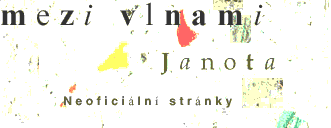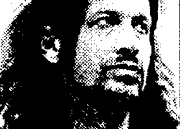:TEXTY .::..::. .::..::. .::..::.N O V I N K Y K O N C E R T Y T E X T Y Č L Á N K Y O B R Á Z K Y V Z K A Z Y L I N K Y K O N T A K T